Solving Linear Equations MathBitsNotebook.com Terms of Use   Contact Person: Donna Roberts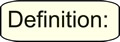An equation states that numerical expressions or algebraic expressions are equal. Linear equations are equations of degree one, where the variable's exponent is one.
 If you need to review your skills on solving simple equations see Review Solving One-Step Equations. Remember: Solving a linear equation is a process of undoing operations that have been applied to the variable when the equation was created. Your goal is to isolate the variable on one side of the equal sign. Remember: You must always make the same changes to BOTH sides of the equal sign to "balance the equation".   Let's take a look at the types of equations we may see: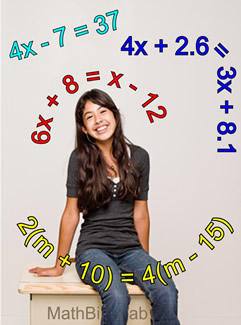Solving two-step equations:
Solve for x   4x - 7 = 37

Analysis: More than one operation has been applied to the variable (4 has been multiplied and 7 has been subtracted). We need to undo these operations in the correct order.
First, isolate the "term" containing the x. Get 4x by itself by
Next, undo the multiplication by 4, by
dividing both sides by 4.
This 2-step process is (1) using the additive inverse property to create a 0 on the left side (-7 + 7 = 0), and using the additive identity to isolate the 4x, since 4x - 0 = 4x.
Then the process (2) is using the multiplicative inverse to create a value of 1 and the multiplicative identity to isolate x.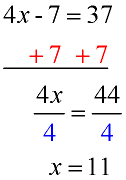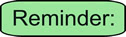Undo any addition or subtraction first, then undo multiplication or division. Just remember that we never divide by zero.
Check answer: A nice bonus to solving equations is that you always know if you have the correct answer. Simply substitute your answer into the original equation and see if the result is true.
Check:
4x - 7 = 37
4(11) - 7 = 37
37 = 37 True!When solving an equation, if your x's drop out and you end up with a statement like 12 = 12, then your equation is an identity and ALL values make it true. If you end up with a statement like 1 = 2, then your equation is a contradiction and NO values make it true. Read more about Truth Values of Equations.Solving equations with variables on both sides of the equal sign:
Solve for x   6x + 8 = x - 12

Analysis: This equation has variables on both sides of the equal sign. We need to get the x's combined into one term.

"Move" the variable with the smaller coefficient (in this case the x term on the right). The sign in front of this x-term is implied to be positive.

Subtract x from both sides.

Now, proceed as shown in the example above.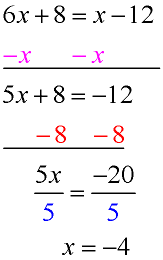When like-variables are scattered throughout a problem, you must combine the like-variables before you will be able to solve the problem.
Check:
6x + 8 = x - 12
6(-4) + 8 = (-4) - 12
-24 + 8 = -4 - 12
-16 = -16 True!Rather than showing all of the steps in solving an equation, some students simply think of "moving" terms across the equal sign. If you think of equations in this manner, remember that moving any term across the "equal sign" changes the term's sign.Solving equations containing parentheses:
Solve for m   2(m + 10) = 4(m - 15)

Analysis: This equation contains parentheses.

The first step is to distribute across the parentheses to get rid of the parentheses. (Multiply through.)

Now, proceed as shown in the examples above.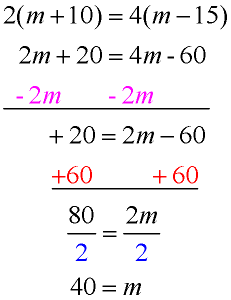When parentheses are involved in the solution of an equation, remember the distributive property.
Check:
2(m + 10) = 4(m - 15)
2(40 + 10) =4(40 - 15)
2(50) = 4(25)
100 = 100 True!If you see an equation like 5x - (3x + 2) = 14, remember that there is an implied "1" in front of the parentheses. 5x - 1(3x + 2) = 14 Distribute "-1", being careful of the signs. 5x - 3x - 2 = 14 (the solution is x = 8.)Solving equations containing decimals:
 Solve for x:    4x + 2.6 = 3x + 8.1 Analysis: Equations may contain decimals as constants (as seen here) or as coefficients. There is no secret to solving problems with decimals. Simply solve as you would solve an equation with integer values. Proceed as shown in the examples above.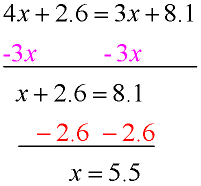Check answer: Check: 4x + 2.6 = 3x + 8.1 4(5.5) + 2.6 =3(5.5) + 8.1 22 + 2.6 =16.5 + 8.1 24.6 = 24.6 True!When solving equaitons with decimals, you can multiply all terms by a power of 10 that will remove the decimal point from the problem. Be sure you remove any parentheses from a problem before attempting this approach. Be sure to multiply carefully. 10 • 4x +10 • 2.6 = 10 • 3x + 10 • 8.1 40x + 26 = 30x + 81 ; 10x = 55 ; x = 5.5Solving equations containing fractions will be discussed in the section
Rational Expressions and Equations.For calculator help with Solving Equations click here.